# Stability and Bifurcation

In linear dynamics, one seeks the fundamental solutions from which one can build all other solutions. In nonlinear dynamics, the main questions are: What is the qualitative behavior of the system? Which and how many non-wandering sets (i.e. a fixed point, a limit cycle, a quasi-periodic or chaotic orbit) occur? Which of them are stable? How does the number of non-wandering sets change while changing a parameter of the system (called control parameter)? The appearance and disappearance of a non-wandering set is called a bifurcation. Change of stability and bifurcation always coincide. There are the two different sides of the same medal!

## 1. Stability

A non-wandering set may be stable or unstable. There are two types of stability, a weaker and a stronger one:

Lyapunov stability:
Every orbit starting in a neighborhood of the non-wandering set remains in a neighborhood.
Asymptotic stability:
In addition to the Lyapunov stability, every orbit in a neighborhood approaches the non-wandering set asymptotically.

Thus, a non-wandering set is either asymptotically stable, marginally stable (i.e. only Lyapunov stable), or unstable. Asymptotically stable non-wandering sets are also called attractors. The basin of attraction is the set of all initial states approaching the attractor in the long time limit.

How can we check the stability of a non-wandering set? There is a scheme called linear stability analysis: Assuming u0(t) is the orbit you want to check whether it is stable or not. It is asymptotically stable if any infinitesimal small perturbations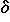u decays. Thus, make the ansatz u = u0+u, put it into the dynamics, i.e., du/dt = F(u) for a time-continuous system, un+1 = F(un) for a time-discrete system, and drop quadratic and higher order terms inu. You will get

du/dt = DF(u0)u

andun+1 = DF(u0)un,

respectively. These linear equations of motion are justified as long as the orbit doesn't go too far away from u0. For fixed points, the fundamental solutions are of the form exp(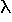st)us and (s)nus, resp., wheres andus (s = 1, 2, ..., dimension of the phase space) are the eigenvalues and eigenvector, respectively, of the Jacobian DF(u0). The eigenvaluess are the roots of the characteristic polynomial det(DF(u0)-)=0. The fixed point u0 is asymptotically stable if all eigenvaluess are inside a stability area of the complex plane. In the time-continuous case, this stability area is the half-plane left of the imaginary axis, whereas in the time-discrete case it is the unit circle around the origin. If at least one eigenvalue is outside the stability area the corresponding fundamental solution would increase exponentially. Thus, the fixed point will be unstable. If an eigenvalue is just on the border of stability, you can not decide by linear stability analysis whether the fixed point is unstable, asymptotically or marginally stable.

Calculating the roots of a polynomial analytically is impossible if its order is greater than five, and tedious if the order is greater than two. For time-continuous system, the question on stability can be answered without explicitely calculating the eigenvalues, thanks to the theorem of Routh and Hurwitz. This theorem says that the real parts of all roots of a polynomial are negative if and only if certain conditions are fulfilled which can be easily calculated. This theorem is useful even in the case of a two-dimensional phase space where the characteristic polynomial is quadratic: Both eigenvalues have negative real part if and only if the determinant DF(u0) is positive and the trace of DF(u0) is negative. The figure below shows a classification scheme of the fixed points of two-dimensional phase spaces.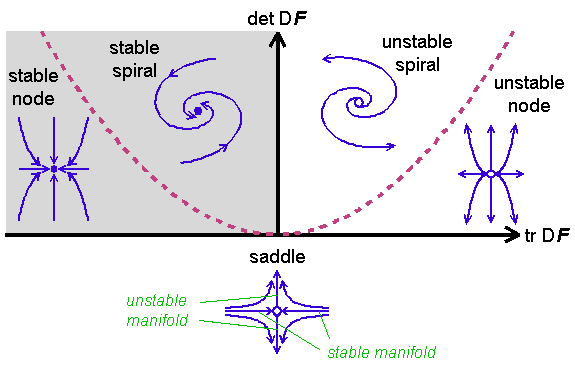The notion spiral and node are inspired by the flow near the fixed point. A pair of conjugated complex eigenvalues lead to a spiral, whereas a node is causes by two real eigenvalues of the same sign. Real eigenvalues of different sign lead to a saddle. In general, a saddle is a fixed point where at least one eigenvalue has a positive real part but also at least one eigenvalue has a negative real part. Near a saddle, an orbit is usually attracted at first but repelled later on. There are points in the phase space which approach the fixed point for t. They form the stable manifold. The eigenspace for the eigenvalues with negative real part is tangential to the stable manifold. The unstable manifold are built by all points approaching the fixed point for t. Saddles and their stable manifolds are usually the boundaries of a basin of attraction. The undriven pendulum has a saddle point (corresponding to the upside-down equilibrium position) and a spiral if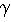< 2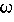0 or a node if> 20 (see the flow on the previous page).

## 2. Bifurcation

The number of attractors in a nonlinear dynamical system can change when a system parameter is changed. This change is called bifurcation. It is accompanied by a change of the stability of an attractor. In a bifurcation point, at least one eigenvalue of the Jacobian gets a zero real part. There are three generic types of so-called co-dimension-one bifurcations (the term codimension counts the number of control parameters for which fine tuning is necessary to get such a bifurcation):In a stationary bifurcation, a single real eigenvalue crosses the boundary of stability. In the Pendulum Lab, stationary bifurcations of limit cycles occur quit often. They are stationary bifuractions of fixed points in a discrete dynamical system because limit cycles are fixed points in the Poincaré map.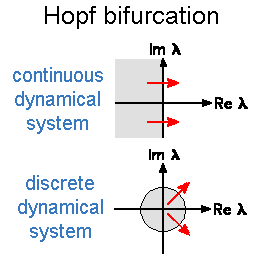Hopf bifurcations occur when a conjugated complex pair crosses the boundary of stability. In the time-continuous case, a limit cycle bifurcates. It has an angular frequency which is given by the imaginary part of the crossing pair. In the discrete case, the bifurcating orbit is generally quasiperiodic, except that the argument of the crossing pair times an integer gives just 2. In the Pendulum Lab, Hopf bifurcations seem to be absent.In discrete dynamical systems, a certain bifurcation is possible which is absent in continuous systems: The period doubling bifurcation. Here, a real eigenvalue crosses the boundary of stability at -1. In a period-doubling bifurcation point, a period-2 orbit bifurcates. You can find this type of bifurcation quite often in the Pendulum Lab.

Crossing the boundary of stability only indicates a bifurcation point and the type of the bifurcating solutions. But it doesn't tell you how and how many new solutions bifurcate or disappear in a bifurcation point. To answer this question one has to take into account the leading nonlinear terms. This might appear to be a difficult task. But fortunately, a theorem and a transformation help us simplifying this analysis:

Center manifold theorem:
It helps to reduce the dimensionality of the phase space to the dimensionality of the so-called center manifold which in the bifurcation point is tangentially to the eigenspace of the marginal modes of the linear stability analysis (i.e., the eigenfunctions which neither decay nor expand exponentially). The center manifold theorem says, the dynamics can be projected onto the center manifold without loosing any significant aspect of the dynamics. Thus, the dynamics near a stationary co-dimension-one bifurcation can be decribed by an effective dynamics in a one-dimensional subspace. Hopf-bifurcations need a two-dimensional phase space. This is exactly the minimum dimension for limit cycles in continuous dynamical systems and for quasiperiodic orbits in discrete dynamical systems. Period-doubling bifurcations need only a one-dimensional phase space.
Transformation to normal form:
The dynamics projected onto the center manifold can by transformed to so-called normal forms by a nonlinear transformation of the phase space variables.

Below, the main normal forms (for continuous dynamical systems) of stationary co-dimension-one bifurcations are shown. The phase space variable is u. The control parameter is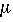. The bifurcation point is at= 0. The direction of motion in the one-dimensional phase space is shown by arrows. Stable (unstable) fixed points are drawn as red solid (dotted) lines. The normal forms are shown in blue.The generic case of stationary co-dimension-one bifurcation is a saddle-node bifurcation. It is generic (that is, the probability is finite to pick a dynamical system having a saddle-node bifurcation) because fixed points lie on a smooth one-dimensional manifold in the combined space of phase space and control parameter. The minima and maxima ofas a function of the curve length denote saddle-node bifurcations where a stable fixed point (a node) annihilates with an unstable one (a saddle in more than one dimension). A combination of a minimum and a maximum lead to the phenomenon of bistability where in a certain interval of the control parameter, two stable attractors exist with an unstable one in-between. Bistability is responsible for hysteresis in many physical and technical systems, as for example the button on your computer mouse. This phenomenon occurs if one sweeps the control parameter relatively slowly beyond the interval of bistability. When the interval of bistability is left the attractor disappears in a saddle-node bifurcation, and the system suddenly jumps to another attractor. For the example of a mouse button, this means that the button makes click even if pressing the button extremely slowly (try it!). Now sweeping the control parameter in the other direction has no effect when the bistability interval is reentered. Thus, the mouse button remains in the pressed state even if the pressure is diminished below the pressure necessary for the previous click. Leaving the bistability interval at its other end, the system has to jump towards the former attractor. And the mouse button makes again click (maybe it sounds different than the first click). In the Pendulum Lab, bistability occurs e.g. in the foldover effect.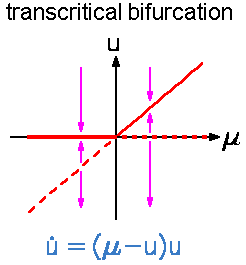The transcritical bifurcation occurs when in the combined space of phase space and controlparameter space two different manifolds of fixed points cross each other. At the crossing point the fixed points exchange there stability property. That is, the unstable fixed point becomes stable and vice versa. Note, that beyond the bifurcation point the number of fixed points has not changed contrary to saddle-node bifurcation where two fixed points appear or disappear. A transcritical bifurcation is not a generic bifurcation in a phase space with more than one dimension because it is unlikely that two lines cross each other in a space with more than two dimensions.Pitchfork bifurcations are possible in dynamical systems with an inversion or reflection symmetry. That is, an equation of motion that remains unchanged if one changes the sign of all phase space variables (or at least for one). An example is the undriven pendulum. Usually, such a system has a symmetric fixed point (or limit cycle). Pitchfork bifurcations are the generic bifurcations when such a symmetric solution changes its stability. The bifurcating solution does not have the full symmetry of the equation of motion. This phenomenon is called broken symmetry. A solution with a broken symmetry does not occur in isolation because the broken symmetry applied onto such a solution generates a new solution where the same symmetry is broken. All such solutions build a family. Therefore, always two fixed points with a broken symmetry bifurcate at once in a pitchfork bifurcation. Both are either stable (supercritical pitchfork bifurcation) or unstable (subcritical pitchfork bifurcation). In the Pendulum Lab, pitchfork bifurcations of limit cycles occur quite often. As an example, try the following experiment: Shake a horizontally driven pendulum faster than its eigen frequency. It will show a tiny oscillation around the equilibrium value= 0. This limit cycle becomes unstable if the driving frequency is much larger than the eigenfrequency. At the bifurcation point, two new stable limit cycles bifurcate supercritically. Their centers are on the right and on the left hand of the unstable symmetric limit cycle.

The bifurcation diagrams of a Hopf and a period doubling bifurcation are similar to the diagram of a pitchfork bifurcation. That is, the bifurcating periodic or quasiperiodic solution is either stable (supercritical bifurcation) or unstable (subcritical bifurcation). Again, a broken symmetry is responsible for this similarity. Here, it is the invariance of the dynamical system against translations in time.

 QUESTIONS worth to think about: Do you know a dynamical system or could you construct one where the stable manifold of a saddle is identical with its unstable manifold? Such an orbit is called a homoclinic orbit. It starts for t-at the saddle and ends for tin the saddle. As mentioned above, a transcritical bifurcation is not a generic bifurcation. What happens with a transcritical bifurcation if you add a small constant to the right hand side of its normal form?

© 1998 Franz-Josef Elmer,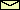Franz-Josef doht Elmer aht unibas doht ch, last modified Sunday, July 26, 1998.